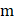# A uniform rod of mass, lengthrests on a smooth horizontal surface. Rod is given a sharp horizontal impulseperpendicular to the rod at a distancefrom the centre. The angular velocity of the rod will be a)b)c)d)## Question ID - 100333 :- A uniform rod of mass, lengthrests on a smooth horizontal surface. Rod is given a sharp horizontal impulseperpendicular to the rod at a distancefrom the centre. The angular velocity of the rod will be a)b)c)d)3537

 (a)Next Question :

Two blocks each of massand a cylinderare connected as shown in Figure. Angular acceleration of the cylinderof radiusis (all strings and pulley are ideal)a)b)c)d)